(ii) Unit Circle The Other Trigonometric Functions
The connection of the other trigonometric functions (secant, cosecant, and cotangent) to the unit circle is also made by use of  "the wrapping function". $P: t \to P(t)= (x(t),y(t))$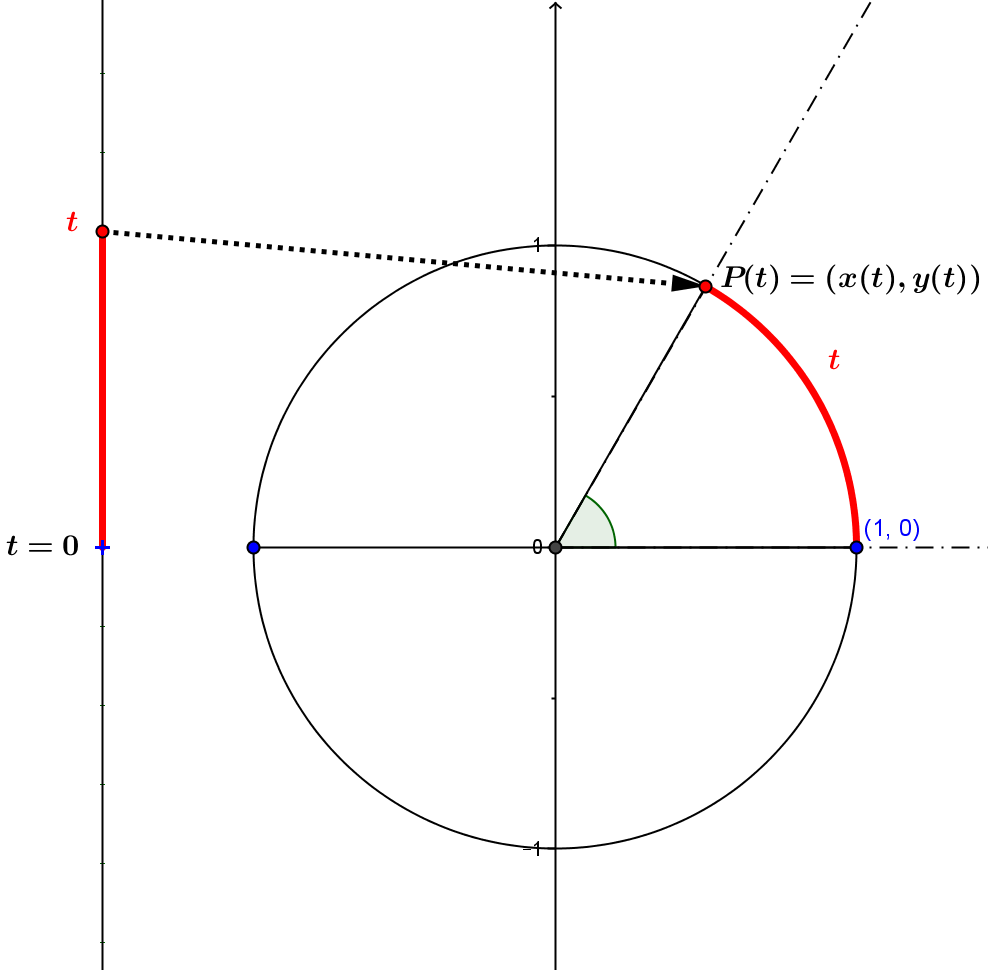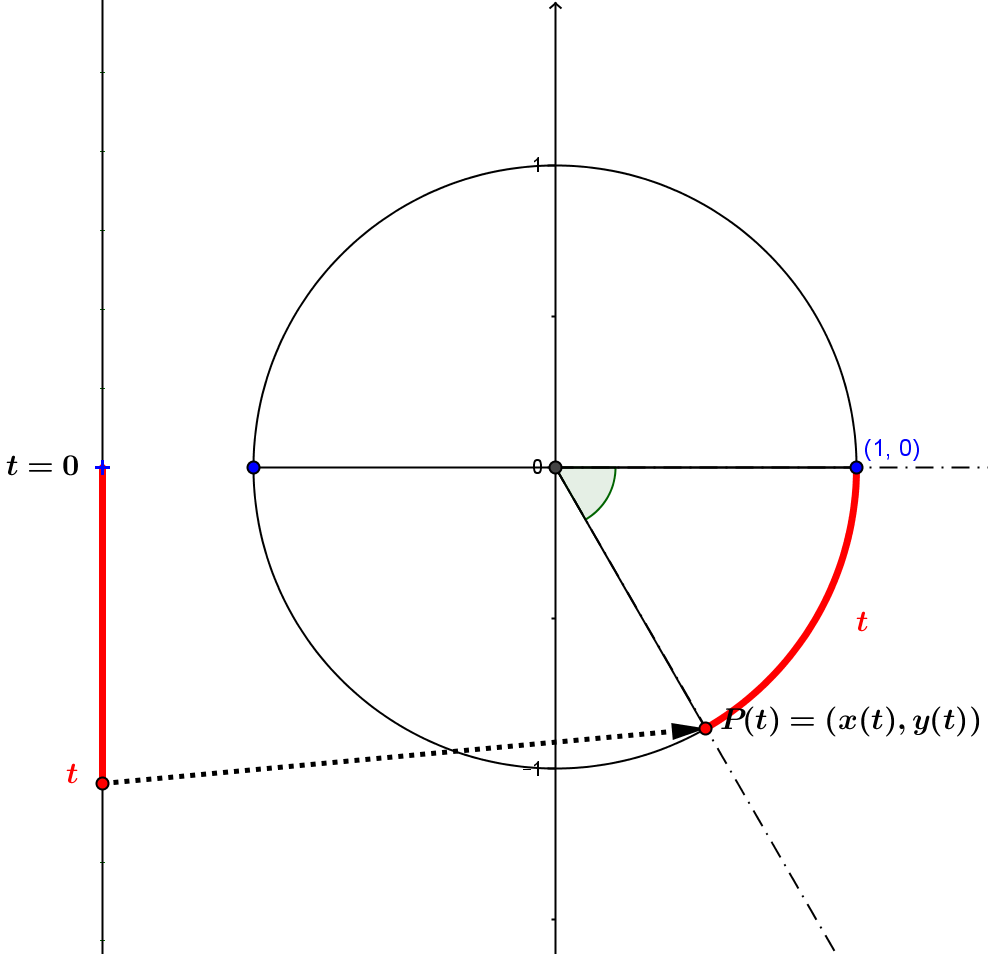After determining the point on the unit circle $P(t)$ by the wrapping function, a projection function based on the coordinates of $P(t)$ onto a line in that plane is used to determine the secant, cosecant, and cotangent function values as follows:

secant: Project $P(t)$ from the point $(0,0)$ to the vertical line $x=1$ to the point $Q(t)=(1, m(t))$ then rotate the segment $OQ(t)$ by $\pi/2 -t$ to the vertical axis to obtain $r_{\pi/2-t}(OQ(t)) = \frac 1{x(t)}= \sec(t)$.
Notes: The secant function is not defined when $x(t) =0$. Thus the domain for the secant function is $Dom_{\sec}=\{ t \in \mathbb R : t \ne (2k+1)\frac\pi 2, k \in \mathbb Z\}$
For $t \in Dom_{\sec}$, we have the identity, $\sec(t)=\frac 1{\cos(t)}$.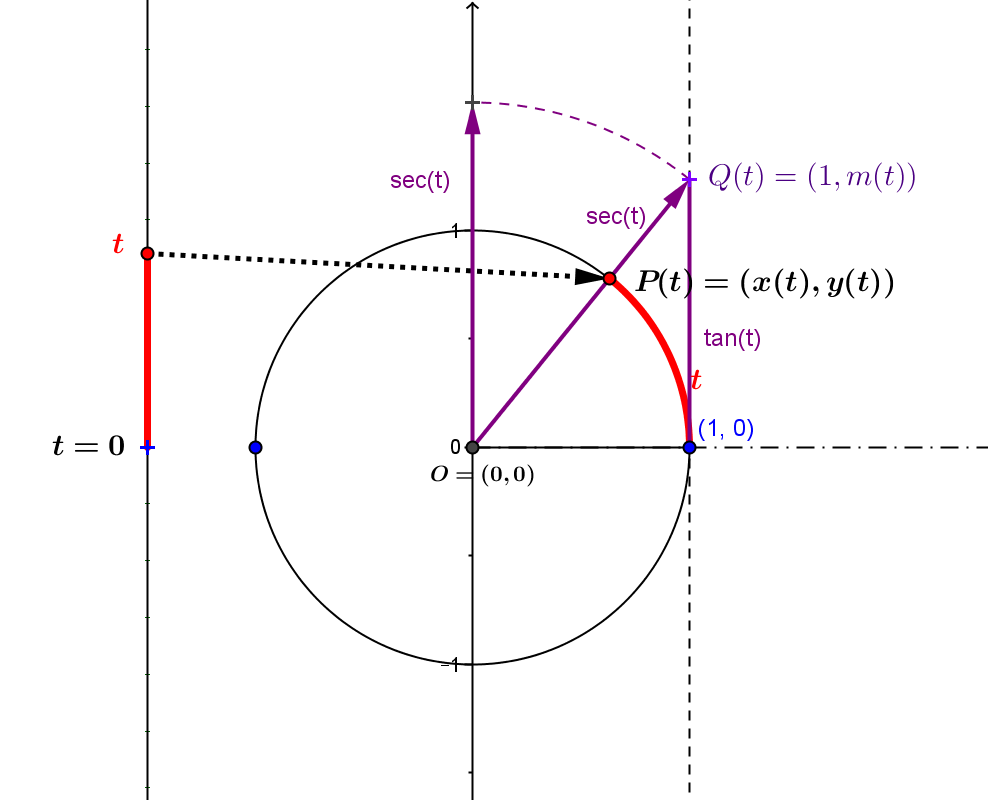cotangent: Project $P(t)$ from the point $(0,0)$ to the horizontal line $y=1$ to the point $(m_c(t),1)$ (applying $pr_{(0,0), (y=1)}$ as the projection function) to obtain $pr_{(0,0), (y=1)}(P(t)) = m_c(t) =\frac {x(t)}{y(t)}= \cot(t)$.
Notes: The cotangent function is not defined when $y(t) =0$. Thus the domain for the cotangent function is $Dom_{cot}=\{ t \in \mathbb R : t \ne k\pi , k \in \mathbb Z\}$
For $t \in Dom_{\cot}$, we have the identity, $\cot(t)=\frac{\cos(t)}{\sin(t)}$.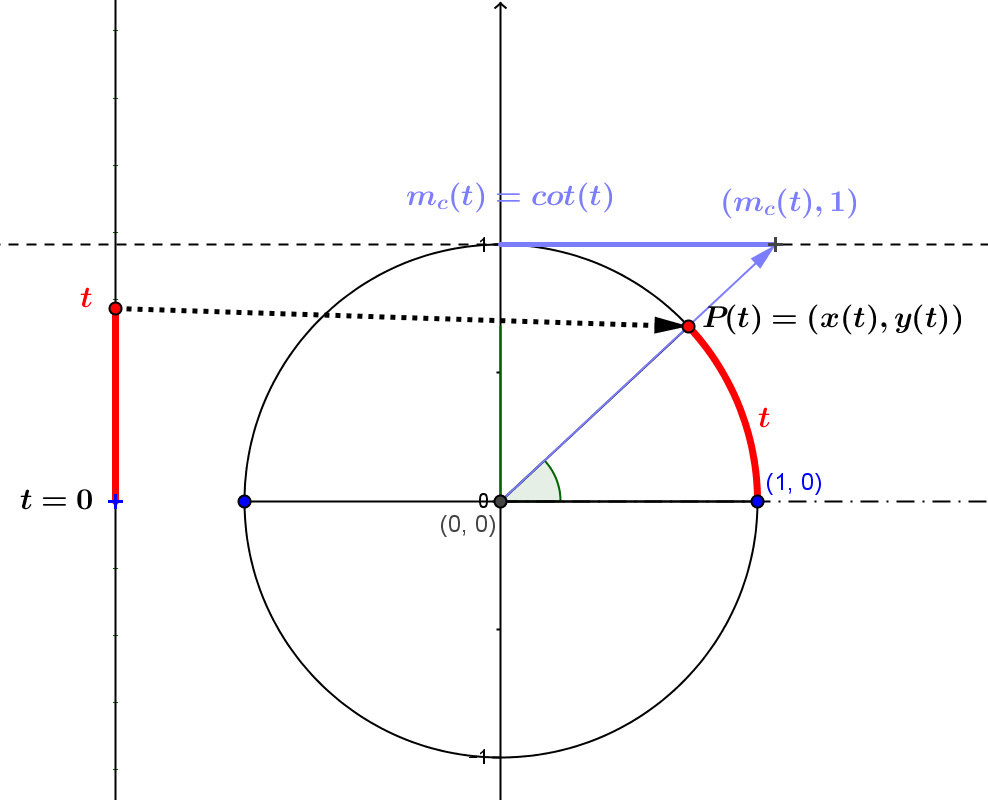cosecant: Project $P(t)$ from the point $O=(0,0)$ to the horizontal line $y=1$ to the point $Q_c(t)=(m_c(t),1)$, then rotate the segment $OQ_c(t)$ by $\pi/2 -t$ to the vertical axis to obtain $r_{\pi/2-t}(OQ_c(t)) = \frac 1{y(t)}= \csc(t)$.
Notes: The cosecant function is not defined when $y(t) =0$. Thus the domain for the cosecant function is $Dom_{\csc}=\{ t \in \mathbb R : t \ne k\pi , k \in \mathbb Z\}$
For $t \in Dom_{\csc}$, we have the identity, $\csc(t)=\frac1{\sin(t)}$.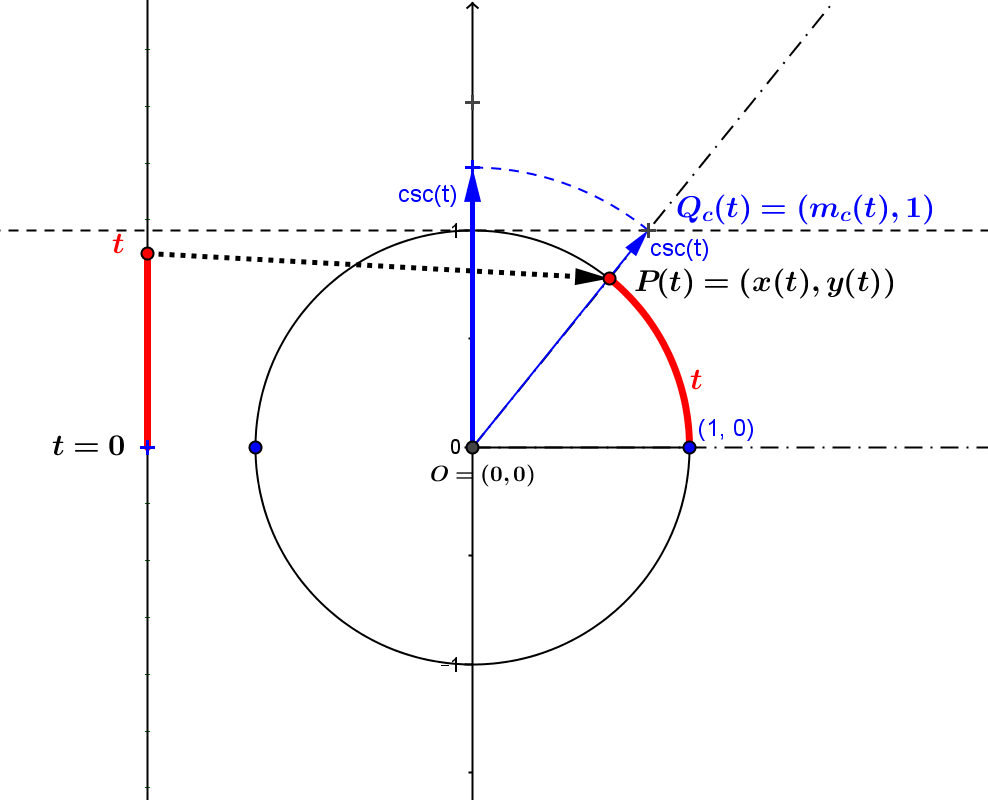Unit Circle Definition of Secant, Cotangent, and Cosecant: GeoGebra Mapping Diagrams

Notice how the mapping diagram values are connected to measurements related to unit circle by considering the line from the origin $O = (0,0)$ and the point $P(t) = (x(t), y(t))$ determined by the wrapping function.
Use the boxes to show or hide one or more of the functions secant, cosecant, or cotangent.
Use the slider to control the angle measured in radians ($-2\pi \le t\le 2\pi$) or degrees ($-360 ^{\circ} \le \Theta \le 360 ^{\circ}$).